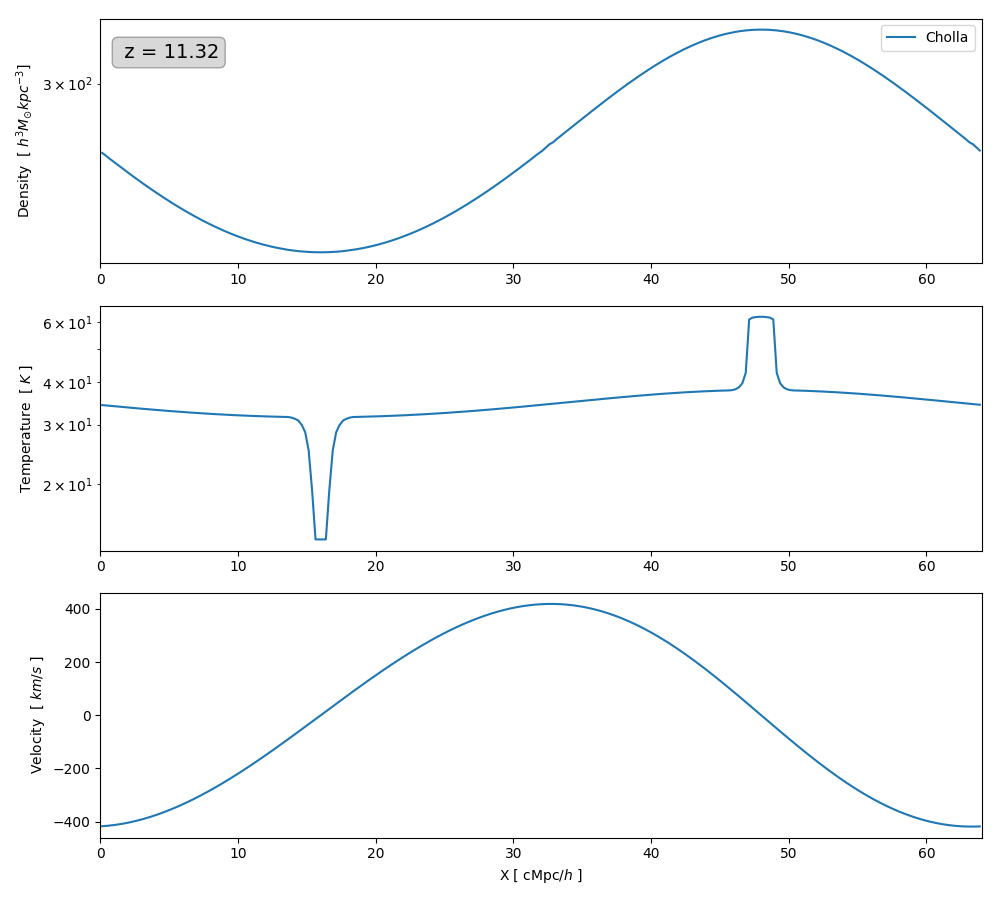There is a Zeldovich Pancake test in Enzo using the next parameters

Lbox = 64 Mpc/h
n_cells = 256
H0 = 50
Omega_m = 1


Setting the initial conditions ( z = 20 ) equal to the ones in Enzo and Using the Only the Advected Internal Energy the evolution is the following :

The evolution of the gas is very similar to enzo until the gas is very compressed and it is not shock heated because I am using the advected internal energy.

Now when I use the Total Internal Energy I get errors in the temperature almost immediately, as you can see below:

The errors are not on the boundary conditions, if I move the density peak from the center, the errors still occur on the maximum and minimum of the density field as you can see below:I conclude that since when using the advected internal energy the results are similar then overall the gravity and cosmology implementation are correct and there is still one error to find in the evolution of the total energy.